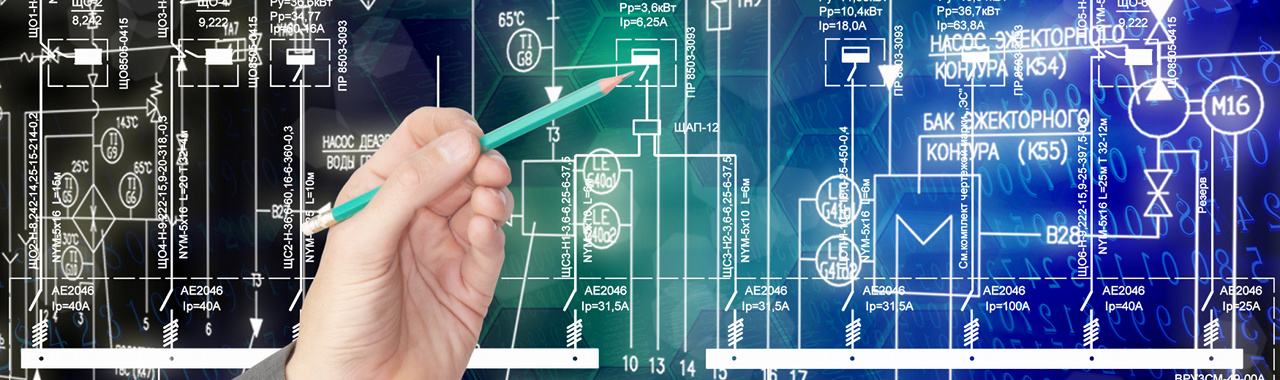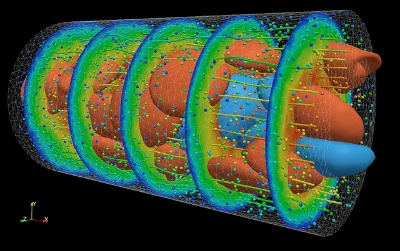# ECE259H1S - Electricity and Magnetism

### Course Description

The fundamental laws of electromagnetics are covered; including Coulomb's law, Gauss’ law, Poisson's and Laplace's equations, the Biot-Savart’s law, Ampere's law, Faraday's law, and Maxwell's equations. Vector calculus is applied to determine the relationship between the electric and magnetic fields and their sources (charges and currents). Field-matter interaction is studied, including polarization in dielectric materials and magnetization in magnetic materials. Circuit elements such as the resistor, capacitor and inductor are introduced from an electromagnetic point of view. Other topics include: electric and magnetic forces, the electric potential, capacitance and inductance, electric and magnetic energy, magnetic circuits, boundary-value problems and transmission-lines.

Online resources

# ECE212 - Circuit Analysis

### Course Description

ECE212 covers fundamental concepts and techniques for the analysis of linear circuits. ECE212 builds on the ECE110 materials and covers:

• The fundamental techniques of circuit analysis (a review of materials from ECE110), i.e., Ohm's law, Kirchhoff's laws, nodal analysis, mesh analysis, superposition, and Thevenin and Norton theorems.

• The linear op-amp and op-amp circuits.

• The transient response of first-order (review of materials from ECE110) and second-order circuits.

• The steady-state response of AC circuits based on the concept of phasors, and steady-state power.

• The frequency response of passive circuits, including the concepts of transfer functions, Bode plots, poles and zeros, resonance, and filters.

• The Laplace transform and solution of circuits in complex-frequency domain.

• Mutually-coupled coils and the ideal transformer

# ECE1254H - Modeling of multiphysics systemsBlood flow simulation (Argonne National Labs)

### Course description

The course deals with the modeling and simulation of physical systems. It introduces the fundamental techniques to generate and solve the equations of a static or dynamic system. Special attention is devoted to complexity issues and to model order reduction methods, presented as a systematic way to simulate highly-complex systems with acceptable computational cost. Examples from multiple disciplines are considered, including electrical/electromagnetic engineering, structural mechanics, fluid-dynamics. Students are encouraged to work on a project related to their own research interests.

### Topics:

• Automatic generation of system equations

• Tableau method

• modified nodal analysis

• Solution of linear and nonlinear systems

• LU decomposition

• sparse systems

• Newton-Raphson method

• Solution of dynamical systems

• Euler and trapezoidal rule

• accuracy, stability

• Model order reduction of linear systems

• proper orthogonal decomposition

• Krylov methods

• truncated balanced realization

• stability/dissipativity enforcement

• Modeling from experimental data

• system identification

• the Vector Fitting algorithm

• enforcement of stability and dissipativity

• If time permits, an overview of numerical methods to solve partial differential equations

• Boundary element method

• finite elements

• FDTD